Courses

# Test: Solid State- 2

## 30 Questions MCQ Test Chemistry Class 12 | Test: Solid State- 2

Description
This mock test of Test: Solid State- 2 for Class 12 helps you for every Class 12 entrance exam. This contains 30 Multiple Choice Questions for Class 12 Test: Solid State- 2 (mcq) to study with solutions a complete question bank. The solved questions answers in this Test: Solid State- 2 quiz give you a good mix of easy questions and tough questions. Class 12 students definitely take this Test: Solid State- 2 exercise for a better result in the exam. You can find other Test: Solid State- 2 extra questions, long questions & short questions for Class 12 on EduRev as well by searching above.
QUESTION: 1

### How many number of atoms are there in a cube based unit cell having one atom on each corner and two atoms on each body diagonal of cube -

Solution:

Total body diagonal = 4
two atoms in each body diagonal so total number of atoms = 4×2=8 and 8 atom on each corner, So its contribution will be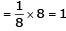so total number of atoms = 8+1=9

QUESTION: 2

### A metal crystallizes with a face-centered cubic lattice. The edge of the unit cell is 408 pm. The diameter of the metal atom is

Solution: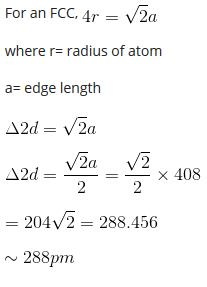QUESTION: 3

### The number of atoms/molecules contained in one face centred cubic unit cell of a mono atomic substance is-

Solution:

In Fcc
Face contribution 1/2
total 6 faces so its contribution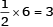atom and contribution by its corner will be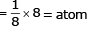So total number of atom in F.C.C will be 4.

QUESTION: 4

In a face centred cubic arrangement of A & B atoms whose A atoms are at the corner of the unit cell & B atoms at the face centres. One of the A atom is missing from one corner in unit cell. The simplest formula of compound is-

Solution:

There are 7 atoms at the corner. so contribution by 7 atoms form corner will be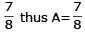B will be at face so total contribution by it will be 3.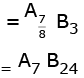QUESTION: 5

Which is/are amorphous solids-

Solution:

Rubber, P lastics and glass, all are amorphous solids so

QUESTION: 6

A solid has a b.c.c. structure. If the distance of closest approach between the two atoms is 1.73 Å. The edge length of the cell is-

Solution:

In B.C.C
4r = a √3
2r = 1.73.
2×1.73 = a √3
Q = 200×10–12 m = 200 pm

QUESTION: 7

In a close packed array of N spheres, the number of tetrahedral holes are-

Solution:

For N spheres, the number of tetrahedral holes is  2N

QUESTION: 8

In a face centred cubic cell, an atom at the face centre is shared by-

Solution: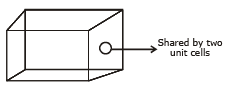QUESTION: 9

A solid XY has NaCl structure. If radius of X+ is 100 pm. What is the radius of Yion-

Solution:

NaCl has octahredral structure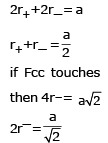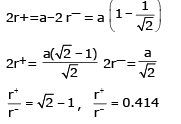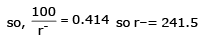QUESTION: 10

How many atoms are there in a unit cell of Mg which forms hexagonal crystals, there being a face-centred atom in each end of the unit cell and 3 completely enclosed atoms within the unit cell-

Solution:

6 face centred atom contbriution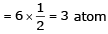and 3 atom and 3 atoms are enclosed so total number of enclosed atom in the unit cell = 3 +3 = 6 atom

QUESTION: 11

A solid is made of two elements X and Z. The atoms Z are in c.c.p. arrangement while atoms X occupy all the tetrahedral sites. What is the formula of the compound-

Solution:

Z is ccp so its total number 4.
X atom occupy tetrahedral void so its total number will be 8
X8Z4 = X2Z

QUESTION: 12

Close packing is maximum in the crystal-

Solution:

Close packing is maximum in either face centrered ork in hexagonal

QUESTION: 13

The vacant space in b.c.c. unit cell is-

Solution:

B.C.C. has the packing efficieny is 68%
so the vacant space in BCCk is 32%

QUESTION: 14

AB crystallizes in a body centred cubic lattice with edge length 'a' equal to 387 pm. The distance between two oppositely charged ions in the lattice is

Solution: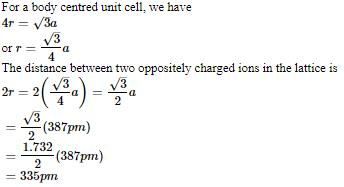option B

QUESTION: 15

The unit cell cube length for LiCl (just like NaCl structure) is 5.14 Å. Assuming anion-anion contact, the ionic radius for chloride ion is-

Solution:

a = 5.14 A°
structure is like NaCl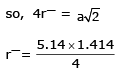r = 1.815 A°

QUESTION: 16

At room temperature, sodium crystallizes in a body centred cubic lattice with a = 4.24 Å. The theoretical density of sodium (At. wt. of Na = 23) is-

Solution: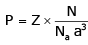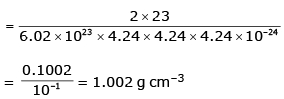QUESTION: 17

The number of octahedral void(s) per atom present in a cubic close-packed structure is

[CBSE AIPMT 2012]

Solution:

The total number of octahedral void(s) per atom present in a cubic close packed structure is 4. Besides the body centre, there is one octahedral void at the centre of each of the 12 edges. It is surrounded by six atoms, four belonging to the same unit cell (2 on the corners and 2 on face centres) and two belonging to two adjacent unit cells. Since each edge of the cube is shared between four adjacent unit cells, so is the octahedral void located on it. Only 1/4​th of each void belongs to a particular unit cell. Thus, in cubic close packed structure, octahedral void at the body-centre of the cube is 1.
12 octahedral voids located at each edge and shared between four unit cells=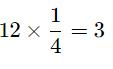Total number of octahedral voids =4
We know that in ccp structure, each unit cell has 4 atoms. Thus, the number of octahedral voids is equal to 4/4=1.

QUESTION: 18

In a face centred cubic lattice the number of nearest neighbour for a given lattice point are-

Solution:

In a face centred cubic lattice the number of nearest neighour for a given lattice point 12

QUESTION: 19

AB crystallizes in a body centred cubic lattice with edge length 'a' equal to 387 pm. The distance between two oppositively charged ions in the lattice is

Solution:

Distance between two oppositely charge ions for BCC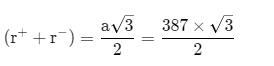= 335.15 pm

QUESTION: 20

A solid AB has rock salt structure. If the edge length is 520 pm and radius of A+ is 80 pm, the radius of anion Bwould be -

Solution: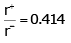putting the value we get 180 pm

QUESTION: 21

A binary solid (A+ B-) has a zinc blende structure with B¯ ions constituting the lattice and A+ ions occupying 25% tetrahedral holes. The formula of solid is-

Solution:

Zns - Zinc blende structure
B- ions consisting the lattice so 4
A here will be 2
A2 B4 = AB2.

QUESTION: 22

A certain metal crystallises in a simple cubic structure. At a certain temperature, it arranges to give a body centred structure. In this transition, the density of the metal-

Solution:

Simple cubic lattice → B.C.C
So the Z will increase
so density will increase

QUESTION: 23

For the structure given below the site marked as S is a-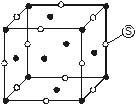Solution:

Centre of edge is octehedral vold so

QUESTION: 24

ZnS is-

Solution:

Zns is ionic erystal

QUESTION: 25

LiF is a/an-

Solution:

LiF iso an ionic crstal

QUESTION: 26

A solid having no definite shape is called-

Solution:

A solid having no definite shape is called Amorphous

QUESTION: 27

The mass of a unit cell of CsCl corresponds to-

Solution:

CsCl has b.c.c. structure
CS+ will be at the centre of the cente of the and 8 Cl will occupy at the corner whch contribution will be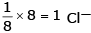lhus, 1 CS+ & 1 Cl

QUESTION: 28

Close packing is maximum in the crystal lattice of-

Solution:

Close packing is maximum in F.C.C.

QUESTION: 29

The structure of MgO is similar to NaCl. The coordination number of Mg is-

Solution:

The correct answer is Option C.

For sodium chloride, there are 6 anions around each cation (coordination number of Na=6) because of the 1:1 stoichiometry, there must also be 6 cations around each Cl-  ion.
Similarly, Mg2+O2- also has the octahedral structure and follows NaCl type geometry.
Therefore, the coordination number of Mg is also 6.

QUESTION: 30

Each unit cell of NaCl consists of 14 chlorine atoms and-

Solution:

12 Na+ will find at centre of the edge and one will at centre of body so 13 Na atoms.